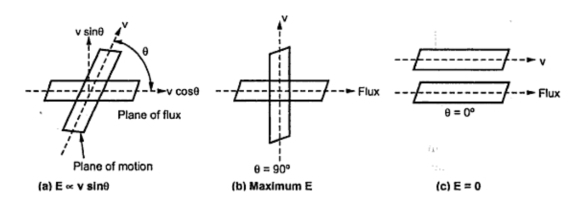If three fingers of a right hand, namely thumb, index finger and middle finger are outstretched so that every one of them is at right angles with the remaining two, and if this position index finger is made to point in the direction of lines of flux, thumb in the direction of the relative motion of the conductor with respect to flux then the outstretched middle finger gives the direction of the e.m.f. induced in the conductor. Visually the rule can be represented as shown in the Fig.1.Fig.  1
This rule mainly gives direction of current which induced e.m.f. in conductor will set up when closed path is provided to it.
Verify the direction of the current through conductor in the four cases shown in the Fig. 2 by using Fleming's right hand rule.Fig. 2
Key Point : It can be observed from the Fig. 2. that if the direction of relative motion of conductor is reversed keeping flux direction same or if flux direction is reversed keeping direction of relative motion of conductor same then the direction of induced e.m.f. and hence direction of current it sets up in an external circuit gets reversed.
The magnitude of the induced e.m.f. is given by,l
E = B x l x v
where          l = Active length of conductor in m.
v = Relative velocity component of conductor in m/s in the direction perpendicular to direction of the flux.
The active length means the length of conductor which is under the influence of magnetic field. In all the cases above, direction of motion of conductor is perpendicular to the plane of the flux.
But if it is not perpendicular then the component of velocity which is perpendicular to the plane of the flux, is only responsible for the induced e.m.f. in the conductor. This is shown in the Fig 3(a). In this Fig. 3(a), though the velocity is v, its component v which is perpendicular to the flux lines is only responsible for the induced e.m.f.
If the plane of the rotation of conductor is parallel to the plane of the flux, there will not be any cutting of flux and hence there can not be any induced e.m.f. in the conductor. This is shown in the Fig.3(b).Fig.  3
Key Point : So to have an induced e.m.f. in the conductor not only the relative motion between the conductor and the flux is necessary but plane of rotation and plane of flux should not be parallel to each other.
If angle between the plane of rotation and the plane of the flux is 'θ' as measured from the axis of the plane of flux then the induced e.m.f. is given by,
E = B l (v sinθ) volts
Where v sinθ is the component of velocity which is perpendicular to the plane of flux and hence responsible for the induced e.m.f. This is shown in the Fig. 4.Fig.  4

From the equation of the induced e.m.f., it can be sen that the basic nature of the induced e.m.f in a d.c. generator is purely sinusoidal i.e. alternating. To have d.c. voltage, a device is used in a d.c. generator to convert the alternating e.m.f. to unidirectional e.m.f. This device is called commutator. An alternator is a machine which produces an alternating e.m.f. without a commutator. So an alternator with a commutator is the basic d.c. generator. Practically there is a difference between the construction of an alternator and a d.c. generator through the basic principle of working is same.